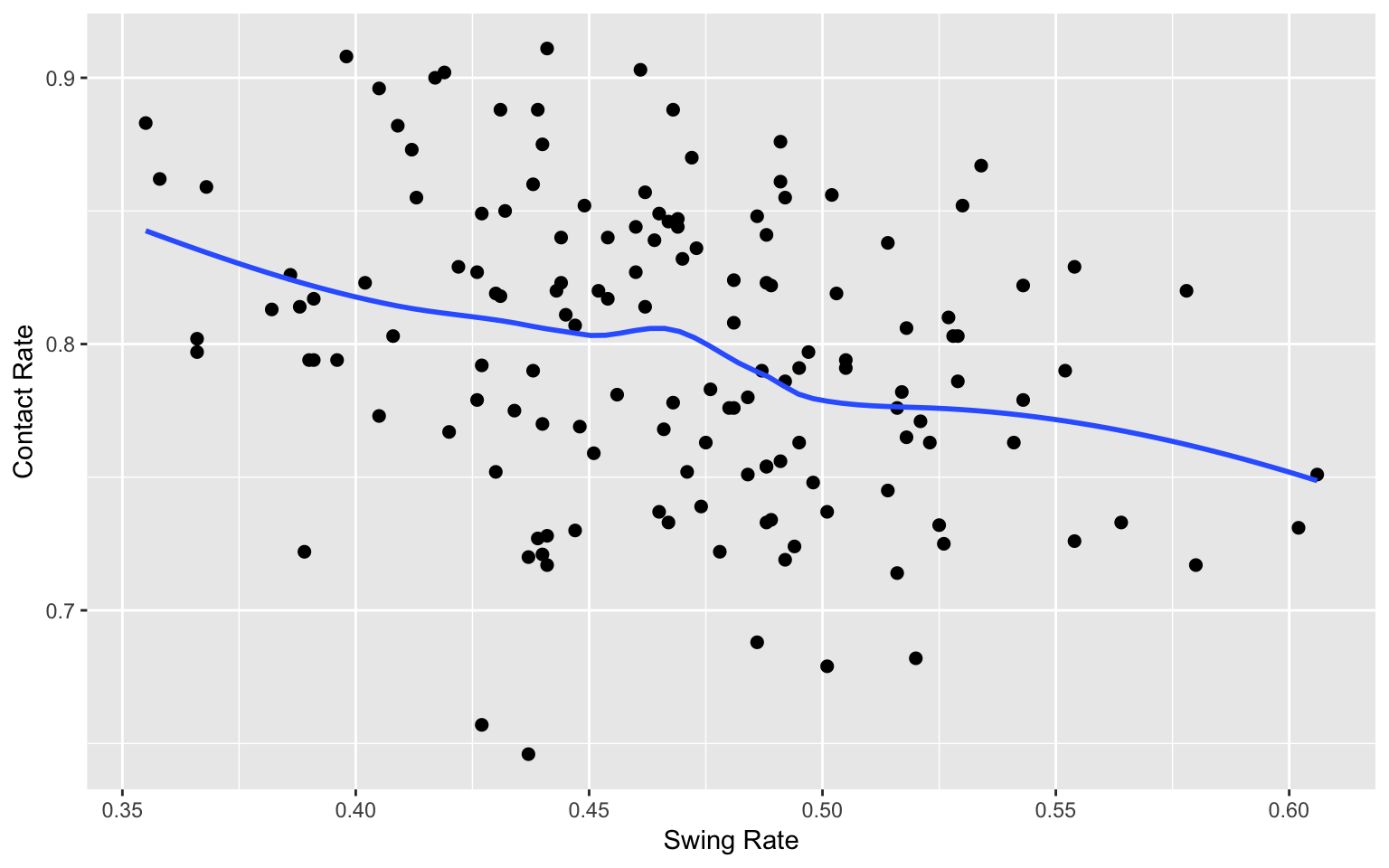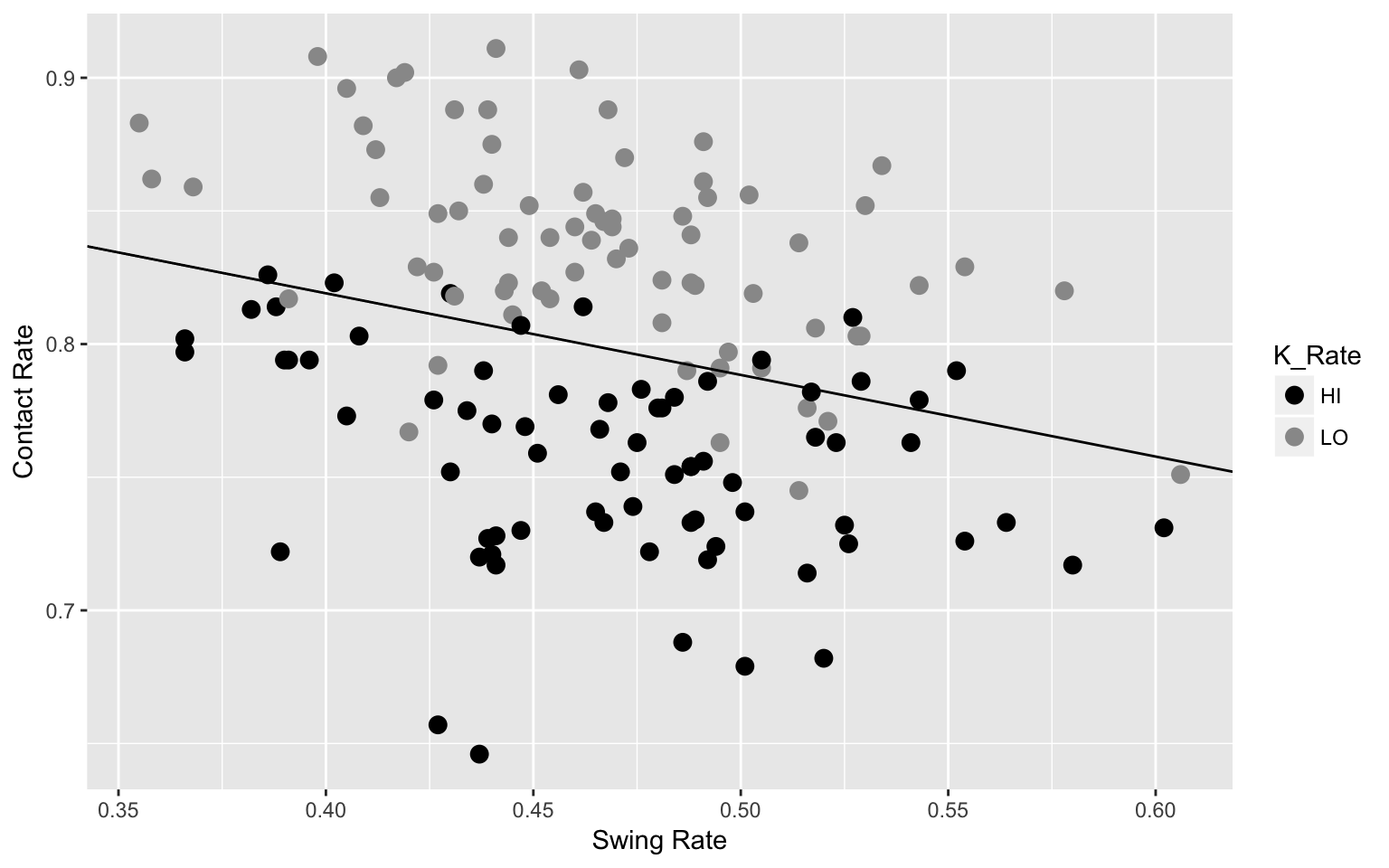### Plate Discipline Statistics for Batters

``````library(tidyverse)
library(ggplot2)``````

#### The Data

Collect several useful tables from Fangraphs. The first dataset contains basic hitting statistics and the second dataset has stats related to plate discipline. We merge the two datasets, creating a single data frame, 146 observations and 33 variables.

``````d1 <- read_csv("https://bayesball.github.io/VB/data/Dashboard_2016.csv")
d <- inner_join(d1, d2, by="playerid")
vars <- c(14, 25:33)
d_subset <- d[, vars]
names(d_subset) <- c("OBP", "O_Swing", "Z_Swing", "Swing",
"O_Contact", "Z_Contact",
"Contact", "Zone",
"F_Strike", "SwStr")
names(d)[c(14, 25:33)] <- names(d_subset)``````

#### Swing and Contact Rates

Following graph constructs a scatterplot of the swing and contact rates for all hitters with a smoothing curve added.

``````ggplot(d, aes(Swing, Contact)) +
geom_point(size=2) +
geom_smooth(se=FALSE) +
xlab("Swing Rate") + ylab("Contact Rate")``````#### Relationship with Strikeout Rate

We divide the players into “high” and “low” strikeout groups. We use contact and swing rates to predict (by a logistic model) the probability a hitter is in the high strikeout group. A line is added to the scatterplot – points above (below) the line are predicted to be in the low (high) K groups.

``````d\$K_Rate <- with(d, ifelse(K > .1875, "HI", "LO"))
d\$y <- ifelse(d\$K_Rate=="HI", 1, 0)
glm(y ~ Contact + Swing, data=d, family=binomial) -> F
ggplot(d, aes(Swing, Contact,
color=K_Rate)) +
geom_point(size=3) +
xlab("Swing Rate") + ylab("Contact Rate") +
geom_abline(intercept = coef(F) / (-coef(F)),
slope = coef(F) / (-coef(F))) +
scale_shape(solid = FALSE) +
scale_colour_manual(values = c("black", "grey60"))``````#### Relationship with Walk Rate

We divide the players into “high” and “low” walk groups. We use contact and swing rates to predict (by a logistic model) the probability a hitter is in the high walk group. A line is added to the scatterplot – points to the left (to the right) of the line are predicted to be in the high (low) walk groups.

``````d\$BB_Cat <- with(d, ifelse(BB > .082, "HI", "LO"))
d\$y <- ifelse(d\$BB_Cat=="HI", 1, 0)
glm(y ~ Contact + Swing, data=d, family=binomial) -> F
ggplot(d, aes(Swing, Contact,
color=BB_Cat)) +
xlab("Swing Rate") + ylab("Contact Rate") +
geom_point(size=3) +
geom_abline(intercept = coef(F) / (-coef(F)),
slope = coef(F) / (-coef(F))) +
scale_shape(solid = FALSE) +
scale_colour_manual(values = c("black", "grey60"))``````